Скачать презентацию TRYGVE HAAVELMO AND THE EMERGENCE OF CAUSAL CALCULUS

• Количество слайдов: 31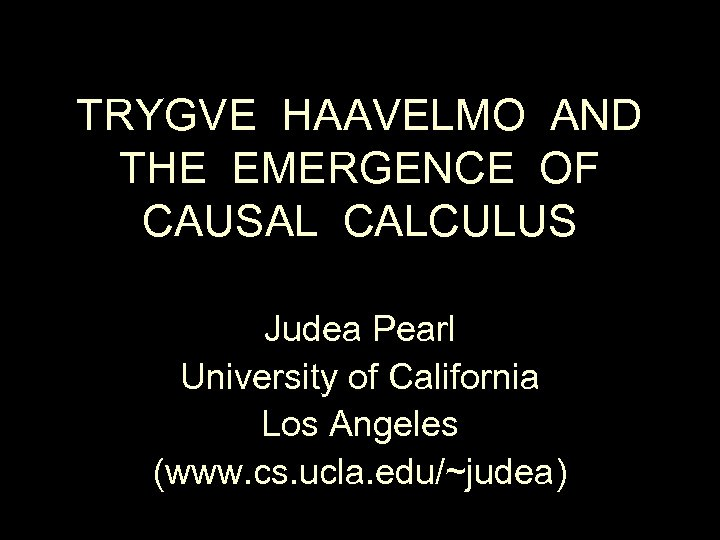TRYGVE HAAVELMO AND THE EMERGENCE OF CAUSAL CALCULUS Judea Pearl University of California Los Angeles (www. cs. ucla. edu/~judea)OUTLINE • Haavelmo’s major breakthroughs • The evolution of causal calculus • What causal calculus can do for us? • Will Haavelmo’s legacy survive?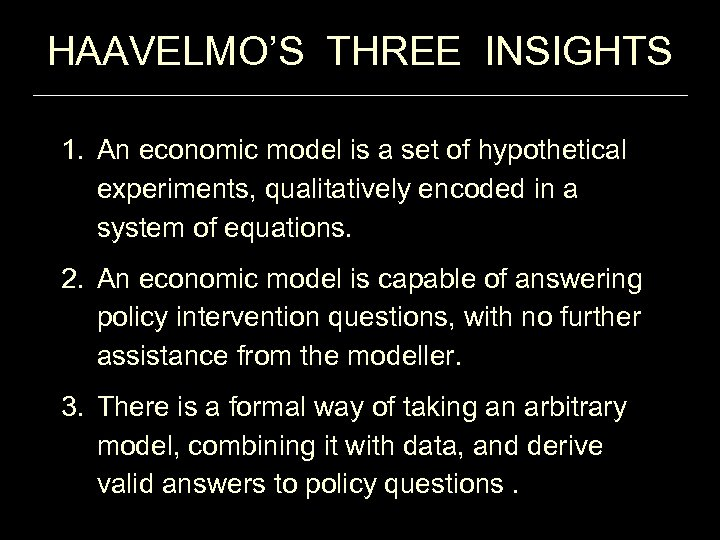HAAVELMO’S THREE INSIGHTS 1. An economic model is a set of hypothetical experiments, qualitatively encoded in a system of equations. 2. An economic model is capable of answering policy intervention questions, with no further assistance from the modeller. 3. There is a formal way of taking an arbitrary model, combining it with data, and derive valid answers to policy questions.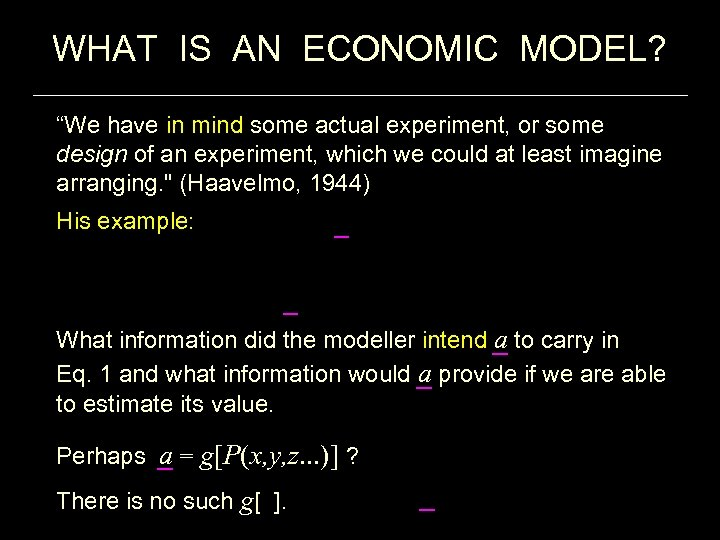WHAT IS AN ECONOMIC MODEL? “We have in mind some actual experiment, or some design of an experiment, which we could at least imagine arranging. " (Haavelmo, 1944) His example: What information did the modeller intend a to carry in Eq. 1 and what information would a provide if we are able to estimate its value. Perhaps a = g[P(x, y, z. . . )] ? There is no such g[ ].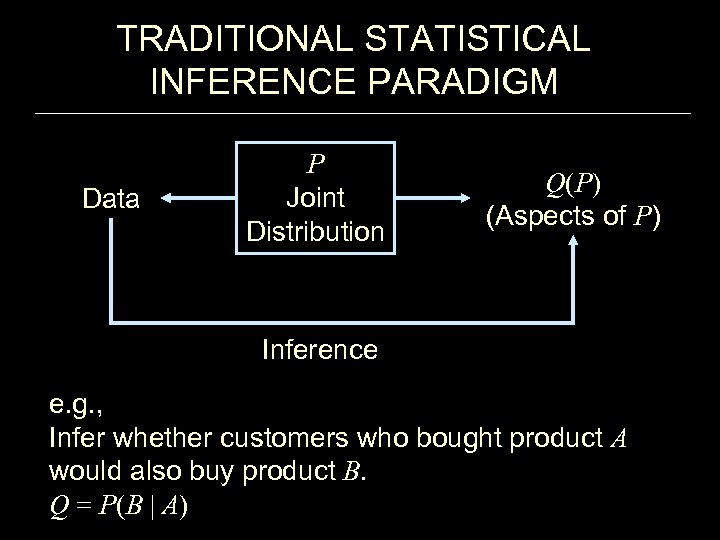TRADITIONAL STATISTICAL INFERENCE PARADIGM Data P Joint Distribution Q(P) (Aspects of P) Inference e. g. , Infer whether customers who bought product A would also buy product B. Q = P(B | A)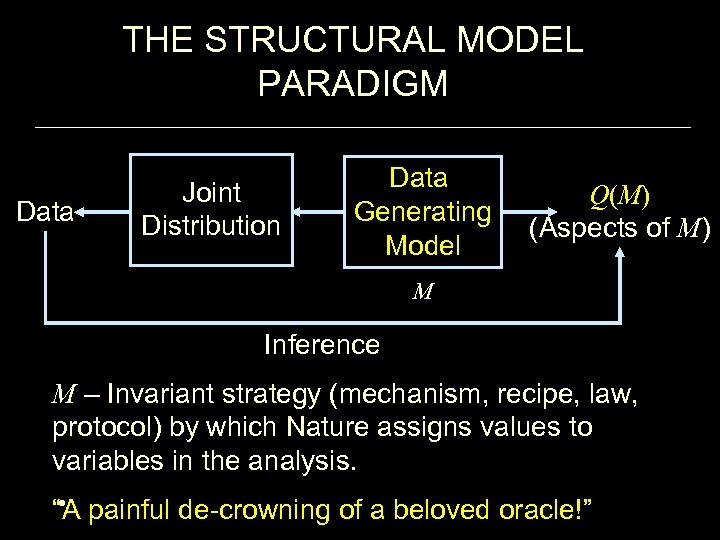THE STRUCTURAL MODEL PARADIGM Data Joint Distribution Data Generating Model Q(M) (Aspects of M) M Inference M – Invariant strategy (mechanism, recipe, law, protocol) by which Nature assigns values to variables in the analysis. • “A painful de-crowning of a beloved oracle!”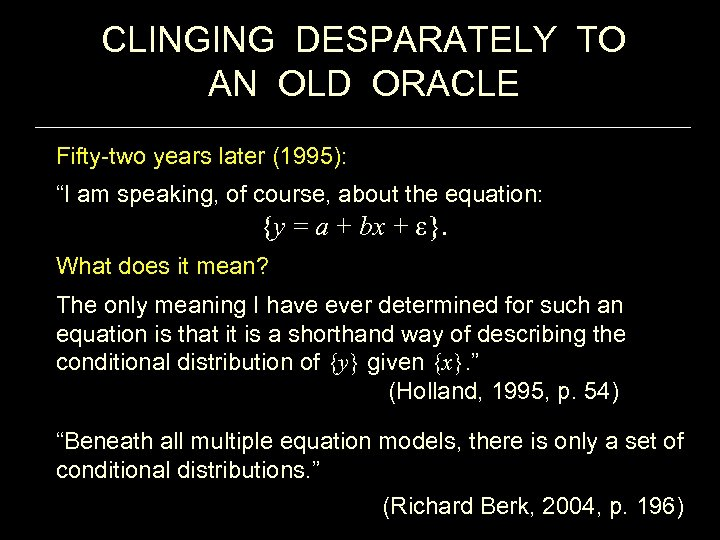CLINGING DESPARATELY TO AN OLD ORACLE Fifty-two years later (1995): “I am speaking, of course, about the equation: {y = a + bx + }. What does it mean? The only meaning I have ever determined for such an equation is that it is a shorthand way of describing the conditional distribution of {y} given {x}. ” (Holland, 1995, p. 54) “Beneath all multiple equation models, there is only a set of conditional distributions. ” (Richard Berk, 2004, p. 196)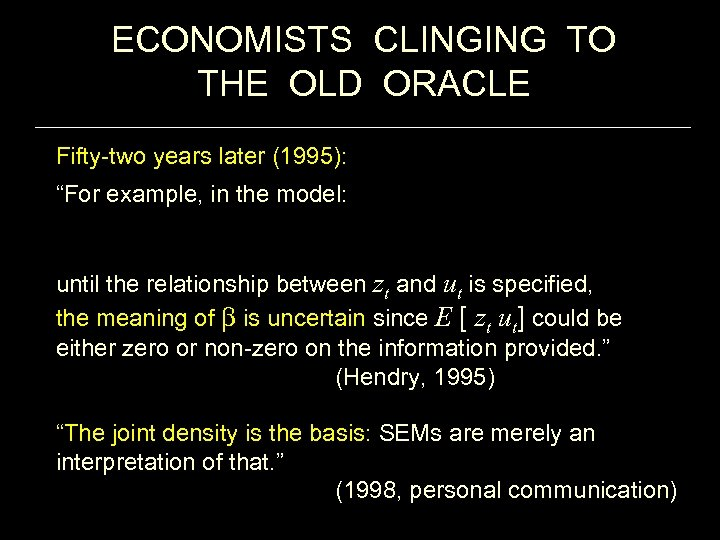ECONOMISTS CLINGING TO THE OLD ORACLE Fifty-two years later (1995): “For example, in the model: until the relationship between zt and ut is specified, the meaning of is uncertain since E [ zt ut] could be either zero or non-zero on the information provided. ” (Hendry, 1995) “The joint density is the basis: SEMs are merely an interpretation of that. ” (1998, personal communication)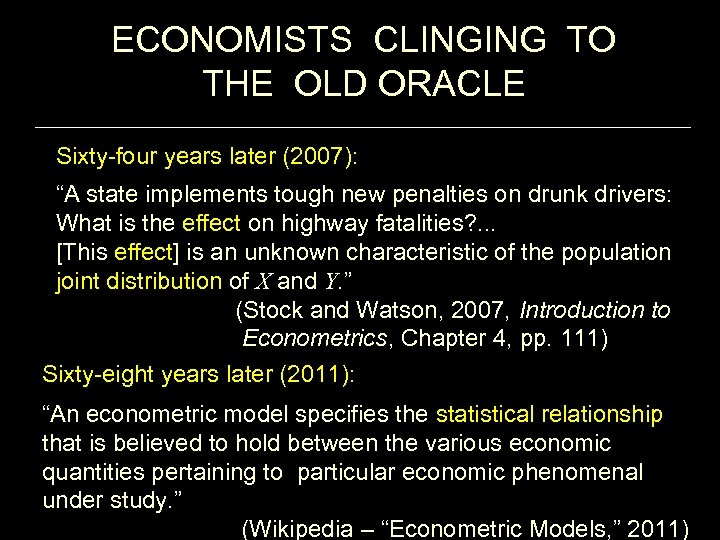ECONOMISTS CLINGING TO THE OLD ORACLE Sixty-four years later (2007): “A state implements tough new penalties on drunk drivers: What is the effect on highway fatalities? . . . [This effect] is an unknown characteristic of the population joint distribution of X and Y. ” (Stock and Watson, 2007, Introduction to Econometrics, Chapter 4, pp. 111) Sixty-eight years later (2011): “An econometric model specifies the statistical relationship that is believed to hold between the various economic quantities pertaining to particular economic phenomenal under study. ” (Wikipedia – “Econometric Models, ” 2011)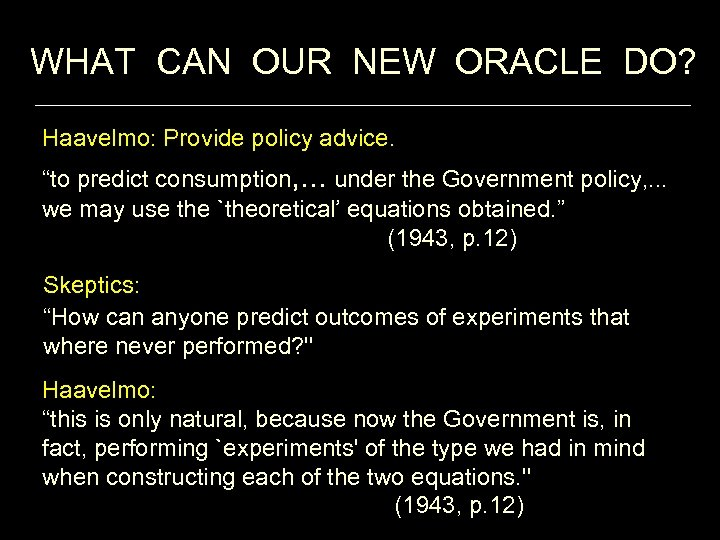WHAT CAN OUR NEW ORACLE DO? Haavelmo: Provide policy advice. “to predict consumption, . . . under the Government policy, . . . we may use the `theoretical’ equations obtained. ” (1943, p. 12) Skeptics: “How can anyone predict outcomes of experiments that where never performed? '' Haavelmo: “this is only natural, because now the Government is, in fact, performing `experiments' of the type we had in mind when constructing each of the two equations. '' (1943, p. 12)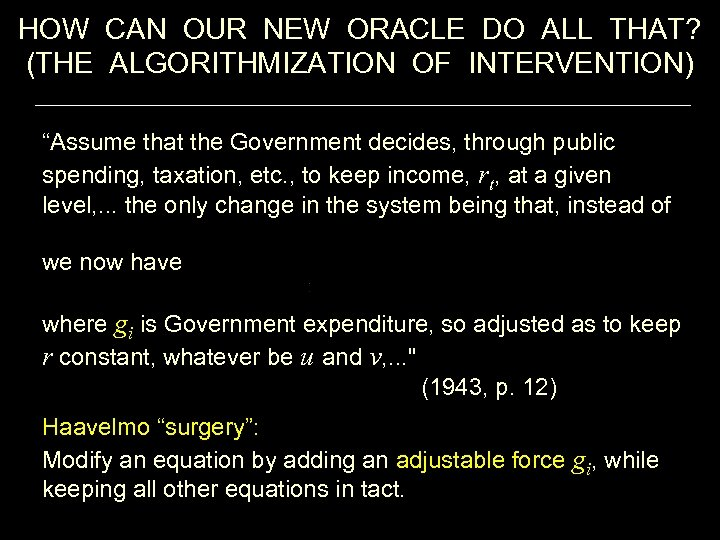HOW CAN OUR NEW ORACLE DO ALL THAT? (THE ALGORITHMIZATION OF INTERVENTION) “Assume that the Government decides, through public spending, taxation, etc. , to keep income, rt, at a given level, . . . the only change in the system being that, instead of we now have where gi is Government expenditure, so adjusted as to keep r constant, whatever be u and v, . . . '' (1943, p. 12) Haavelmo “surgery”: Modify an equation by adding an adjustable force gi, while keeping all other equations in tact.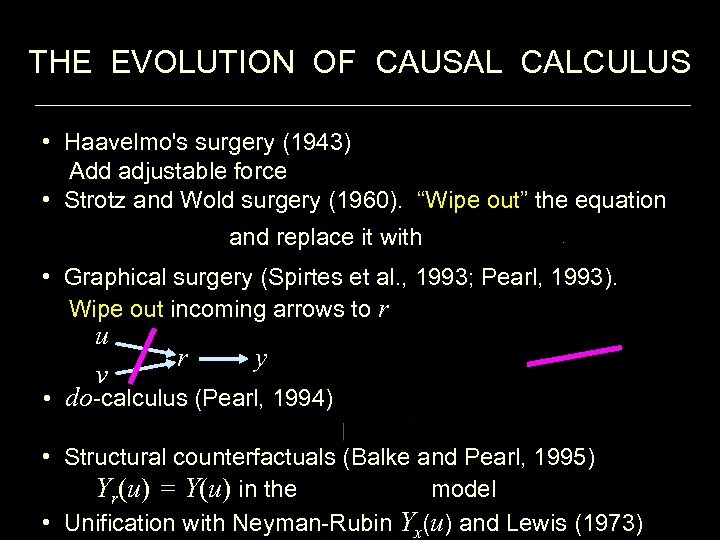THE EVOLUTION OF CAUSAL CALCULUS • Haavelmo's surgery (1943) Add adjustable force • Strotz and Wold surgery (1960). “Wipe out” the equation and replace it with • Graphical surgery (Spirtes et al. , 1993; Pearl, 1993). Wipe out incoming arrows to r u v r y • do-calculus (Pearl, 1994) • Structural counterfactuals (Balke and Pearl, 1995) Yr(u) = Y(u) in the model • Unification with Neyman-Rubin Yx(u) and Lewis (1973)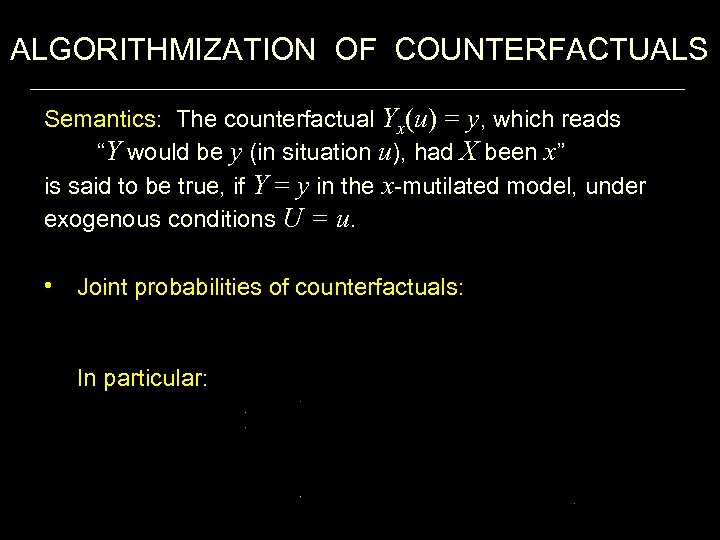ALGORITHMIZATION OF COUNTERFACTUALS Semantics: The counterfactual Yx(u) = y, which reads “Y would be y (in situation u), had X been x” is said to be true, if Y = y in the x-mutilated model, under exogenous conditions U = u. • Joint probabilities of counterfactuals: In particular: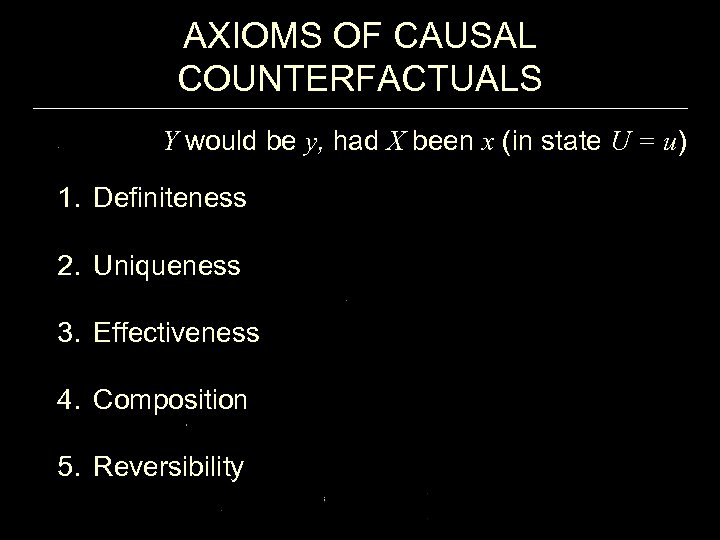AXIOMS OF CAUSAL COUNTERFACTUALS Y would be y, had X been x (in state U = u) 1. Definiteness 2. Uniqueness 3. Effectiveness 4. Composition 5. Reversibility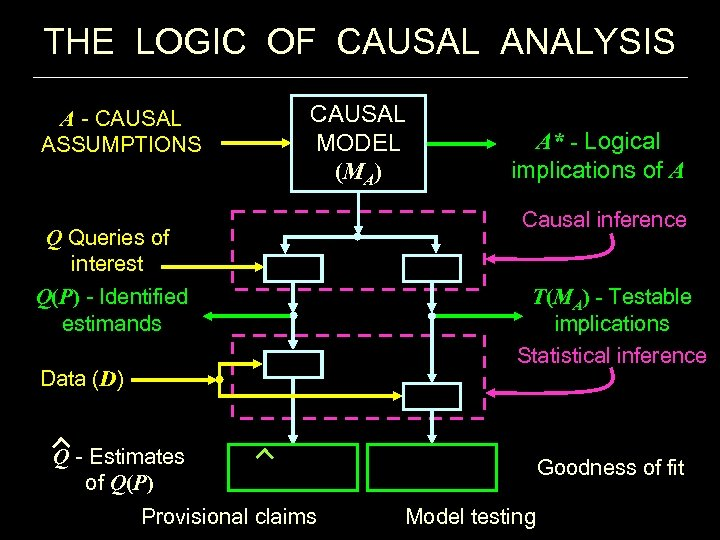THE LOGIC OF CAUSAL ANALYSIS A - CAUSAL ASSUMPTIONS CAUSAL MODEL (MA) Q Queries of interest Q(P) - Identified estimands Data (D) A* - Logical implications of A Causal inference T(MA) - Testable implications Statistical inference Q - Estimates of Q(P) Provisional claims Goodness of fit Model testing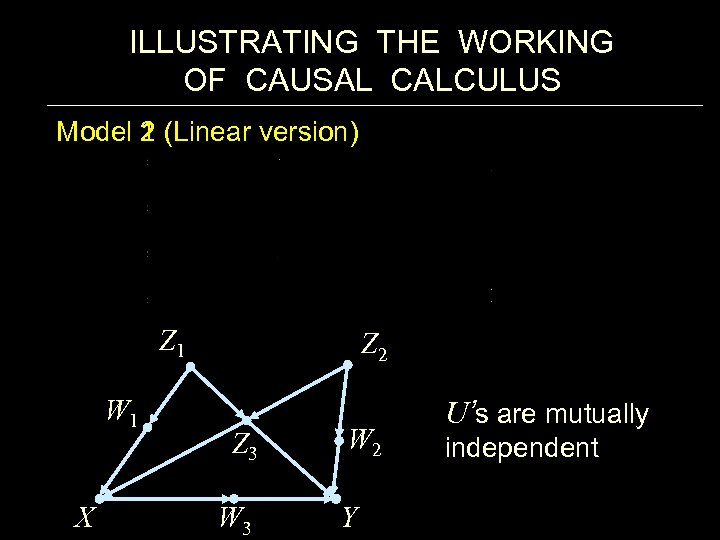ILLUSTRATING THE WORKING OF CAUSAL CALCULUS Model 1 Model 2 (Linear version) Z 1 W 1 X Z 2 Z 3 W 2 Y U’s are mutually independent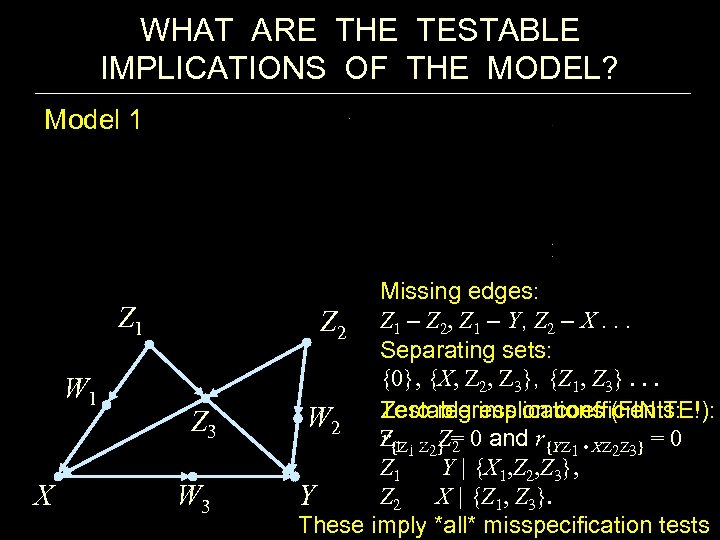WHAT ARE THE TESTABLE IMPLICATIONS OF THE MODEL? Model 1 Z 1 W 1 X Z 3 W 3 Missing edges: Z 2 Z 1 – Z 2, Z 1 – Y, Z 2 – X. . . Separating sets: {0}, {X, Z 2, Z 3}, {Z 1, Z 3}. . . Zero regression coefficients: W 2 Testable implications (FINITE!): r 1 Z 2 Z{Z 1 Z 2} = 0 and r{YZ 1 • XZ 2 Z 3} = 0 Z 1 Y | {X 1, Z 2, Z 3}, Z 2 X | {Z 1, Z 3}. Y These imply *all* misspecification tests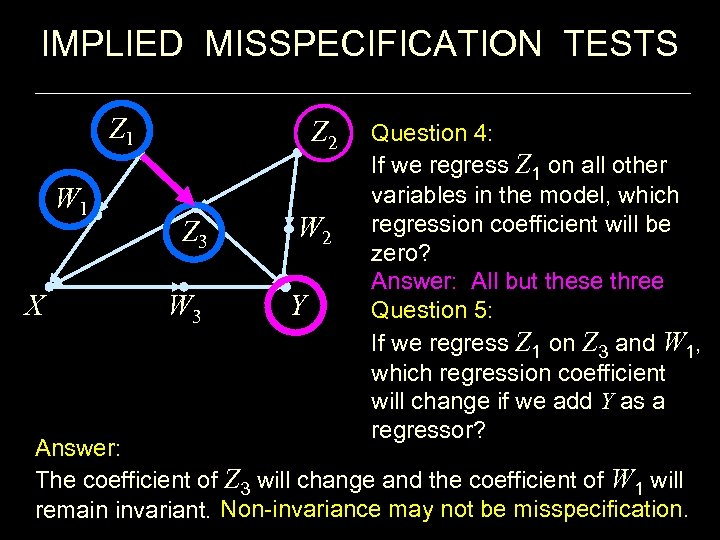IMPLIED MISSPECIFICATION TESTS Z 1 W 1 X Z 2 Z 3 W 2 Y Question 4: If we regress Z 1 on all other variables in the model, which regression coefficient will be zero? Answer: All but these three Question 5: If we regress Z 1 on Z 3 and W 1, which regression coefficient will change if we add Y as a regressor? Answer: The coefficient of Z 3 will change and the coefficient of W 1 will remain invariant. Non-invariance may not be misspecification.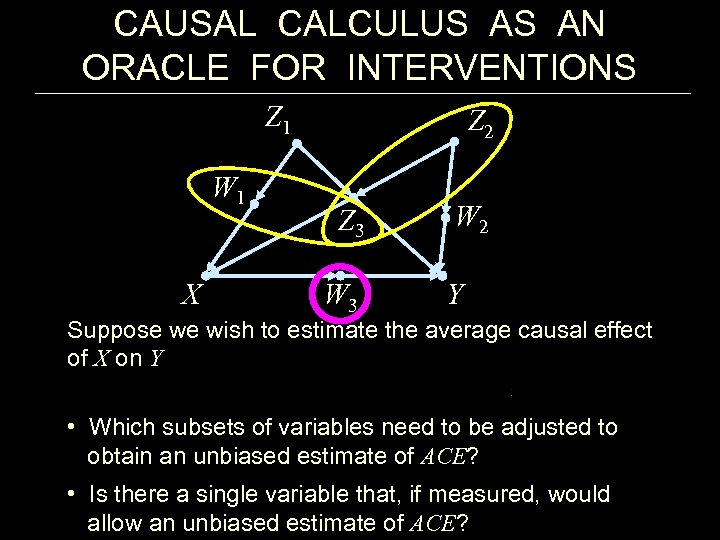CAUSAL CALCULUS AS AN ORACLE FOR INTERVENTIONS Z 1 W 1 X Z 2 Z 3 W 2 Y Suppose we wish to estimate the average causal effect of X on Y • Which subsets of variables need to be adjusted to obtain an unbiased estimate of ACE? • Is there a single variable that, if measured, would allow an unbiased estimate of ACE?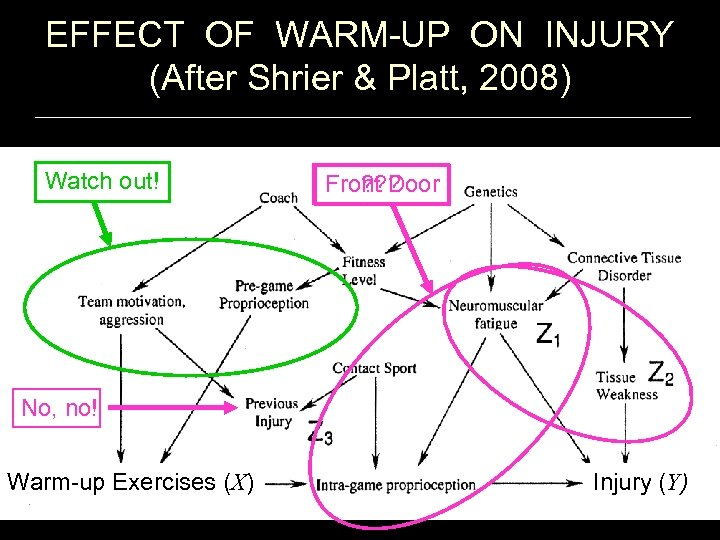EFFECT OF WARM-UP ON INJURY (After Shrier & Platt, 2008) Watch out! ? ? ? Front Door No, no! Warm-up Exercises (X) Injury (Y)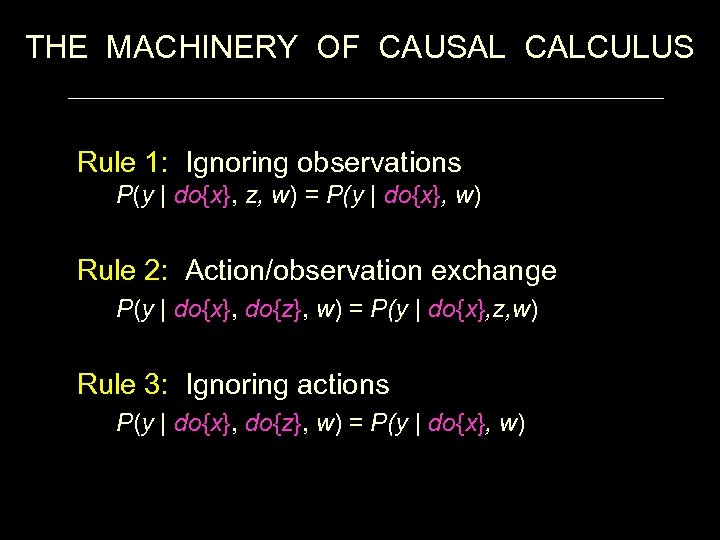THE MACHINERY OF CAUSAL CALCULUS Rule 1: Ignoring observations P(y | do{x}, z, w) = P(y | do{x}, w) Rule 2: Action/observation exchange P(y | do{x}, do{z}, w) = P(y | do{x}, z, w) Rule 3: Ignoring actions P(y | do{x}, do{z}, w) = P(y | do{x}, w)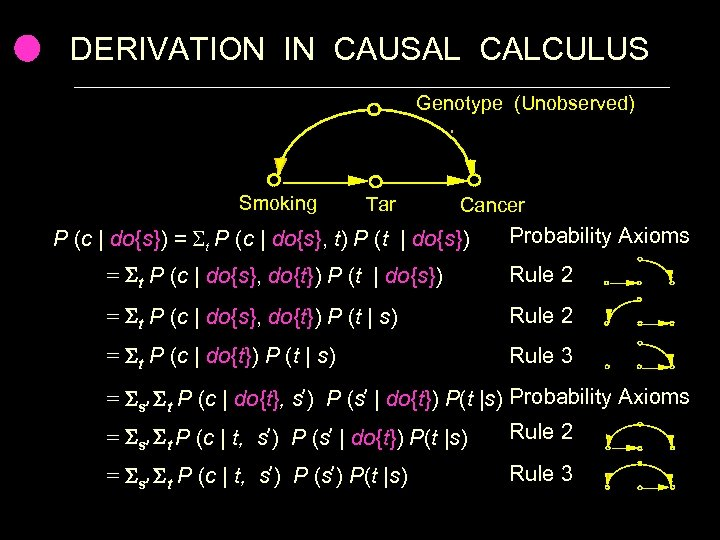DERIVATION IN CAUSAL CALCULUS Genotype (Unobserved) Smoking Tar Cancer P (c | do{s}) = t P (c | do{s}, t) P (t | do{s}) Probability Axioms = t P (c | do{s}, do{t}) P (t | do{s}) Rule 2 = t P (c | do{s}, do{t}) P (t | s) Rule 2 = t P (c | do{t}) P (t | s) Rule 3 = s t P (c | do{t}, s ) P (s | do{t}) P(t |s) Probability Axioms Rule 2 = P (c | t, s ) P (s | do{t}) P(t |s) s t = s t P (c | t, s ) P (s ) P(t |s) Rule 3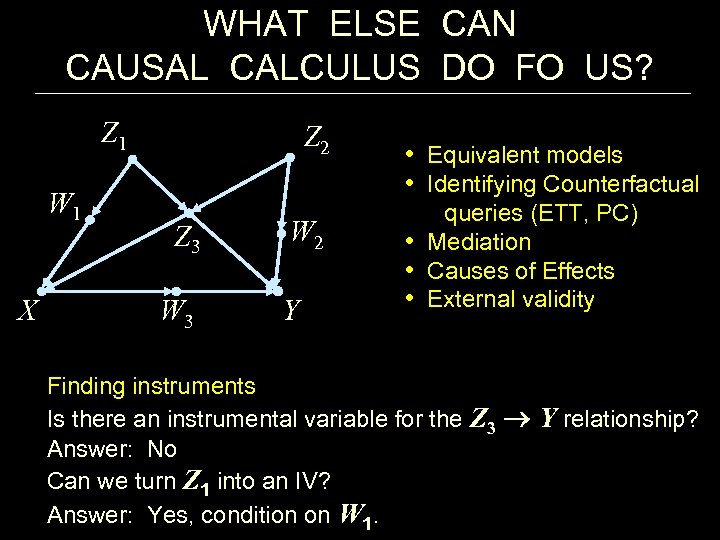WHAT ELSE CAN CAUSAL CALCULUS DO FO US? Z 1 W 1 X Z 2 Z 3 W 2 Y • Equivalent models • Identifying Counterfactual queries (ETT, PC) • Mediation • Causes of Effects • External validity Finding instruments Is there an instrumental variable for the Z 3 Y relationship? Answer: No Can we turn Z 1 into an IV? Answer: Yes, condition on W 1.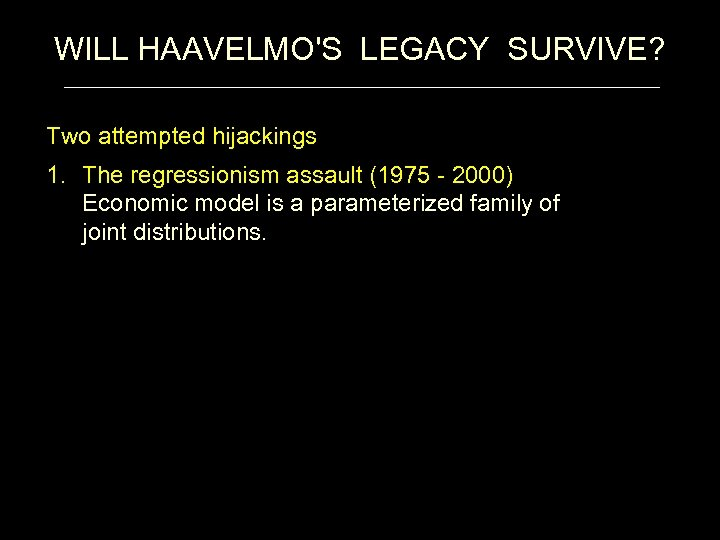WILL HAAVELMO'S LEGACY SURVIVE? Two attempted hijackings 1. The regressionism assault (1975 - 2000) Economic model is a parameterized family of joint distributions.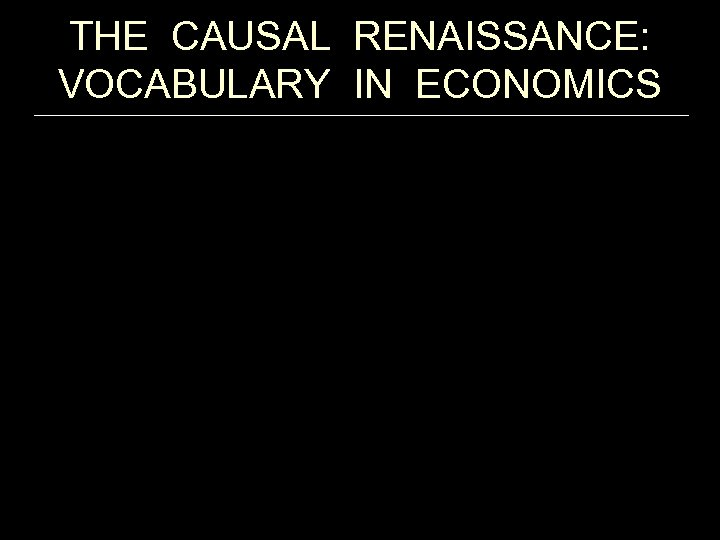THE CAUSAL RENAISSANCE: VOCABULARY IN ECONOMICS From Hoover (2004) “Lost Causes”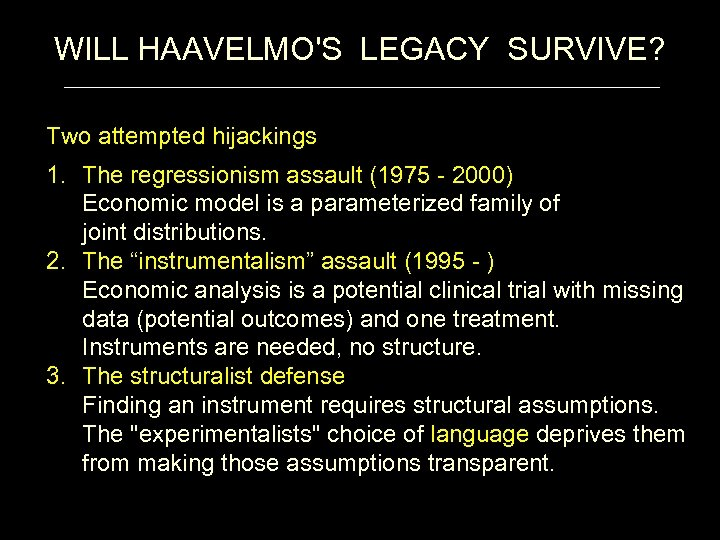WILL HAAVELMO'S LEGACY SURVIVE? Two attempted hijackings 1. The regressionism assault (1975 - 2000) Economic model is a parameterized family of joint distributions. 2. The “instrumentalism” assault (1995 - ) Economic analysis is a potential clinical trial with missing data (potential outcomes) and one treatment. Instruments are needed, no structure. 3. The structuralist defense Finding an instrument requires structural assumptions. The "experimentalists" choice of language deprives them from making those assumptions transparent.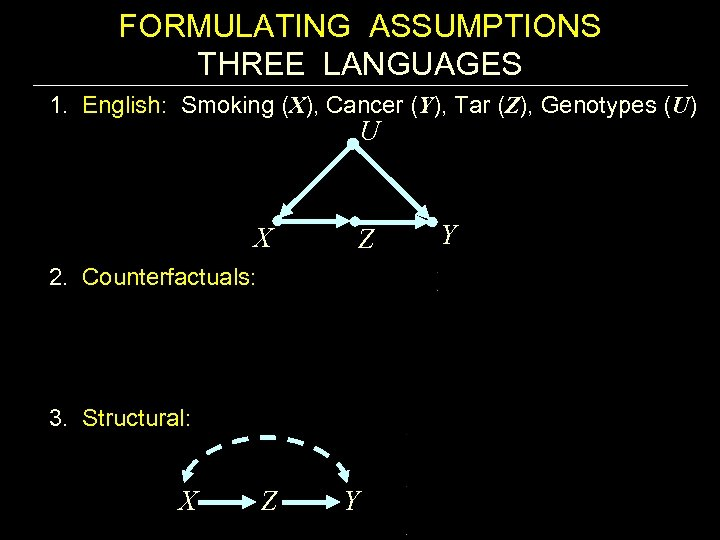FORMULATING ASSUMPTIONS THREE LANGUAGES 1. English: Smoking (X), Cancer (Y), Tar (Z), Genotypes (U) U X 2. Counterfactuals: Z 3. Structural: X Z Y Y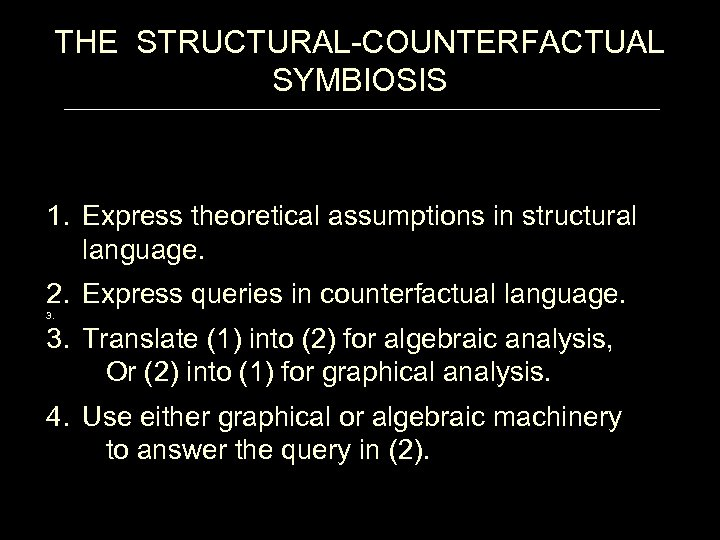THE STRUCTURAL-COUNTERFACTUAL SYMBIOSIS 1. Express theoretical assumptions in structural language. 2. Express queries in counterfactual language. 3. 3. Translate (1) into (2) for algebraic analysis, Or (2) into (1) for graphical analysis. 4. Use either graphical or algebraic machinery to answer the query in (2).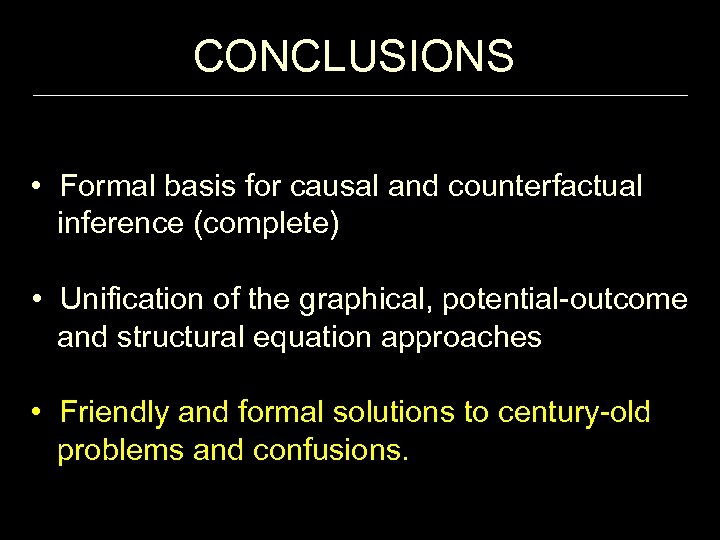CONCLUSIONS • Formal basis for causal and counterfactual inference (complete) • Unification of the graphical, potential-outcome and structural equation approaches • Friendly and formal solutions to century-old problems and confusions.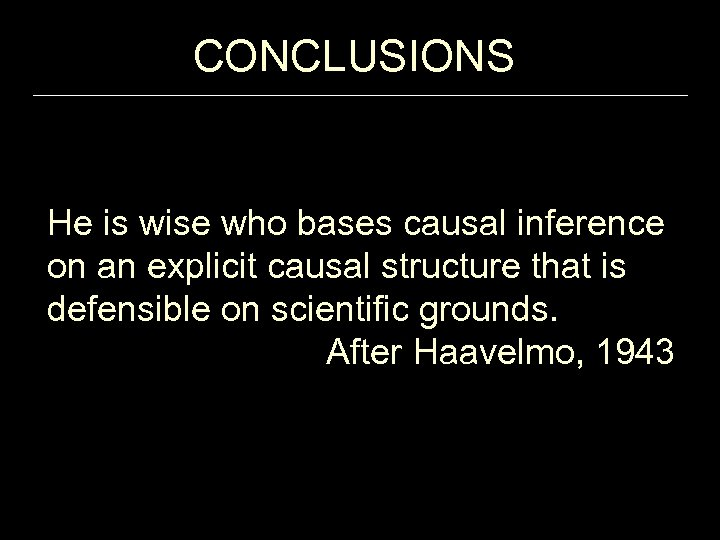CONCLUSIONS He is wise who bases causal inference on an explicit causal structure that is defensible on scientific grounds. After Haavelmo, 1943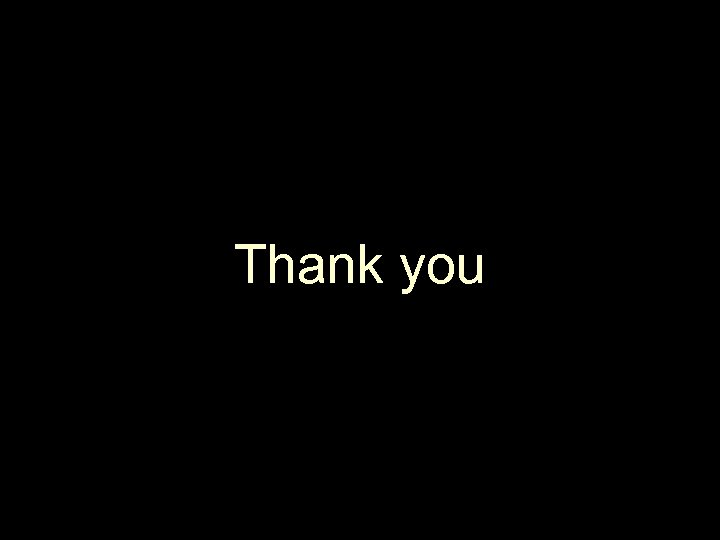Thank you